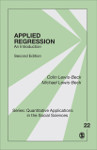# Applied Regression: An Introduction

Known for its readability and clarity, this Second Edition of the best-selling Applied Regression provides an accessible introduction to regression analysis for social scientists and other professionals who want to model quantitative data. After covering the basic idea of fitting a straight line to a scatter of data points, the text uses clear language to explain both the mathematics and assumptions behind the simple linear regression model. The authors then cover more specialized subjects of regression analysis, such as multiple regression, measures of model fit, analysis of residuals, interaction effects, multicollinearity, and prediction. Throughout the text, graphical and applied examples help explain and demonstrate the power and broad applicability of regression analysis for answering scientific questions.

•••